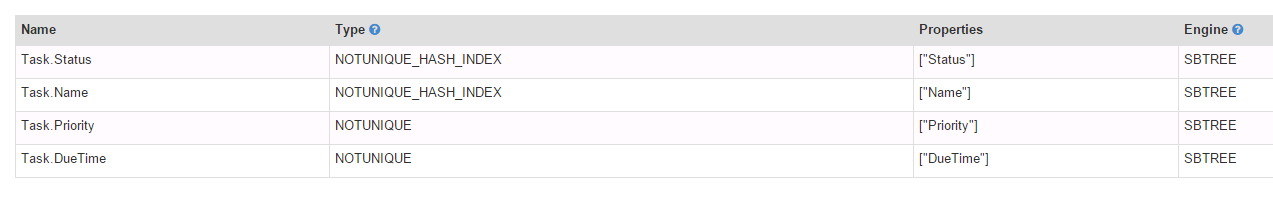How to speed up ORDER BY

191 views

Hung Tran

Apr 25, 2016, 1:14:03 AM4/25/16
Hi,

I am using OrientDB 2.1.8 on Windows 2012 server platform. I have a table about 1.117.549 records, with indices as following.Here are some queries and its timing captured from OrientDB Studio.

SELECT  FROM Task WHERE (((DueTime <= '2016-04-25 04:19:58') AND (Status = 0)) OR ((ProcessId = 1) AND (Status = 10))) AND (EntityInfo.State = 0) ORDER BY Priority DESC, DueTime DESC, @rid ASC limit 1
=> it took 80.978 sec.

SELECT  FROM Task WHERE (((DueTime <= '2016-04-25 04:19:58') AND (Status = 0)) OR ((ProcessId = 1) AND (Status = 10))) AND (EntityInfo.State = 0) ORDER BY Priority DESC, DueTime DESC limit 1
=> it took 81.544 sec.

SELECT  FROM Task WHERE (((DueTime <= '2016-04-25 04:19:58') AND (Status = 0)) OR ((ProcessId = 1) AND (Status = 10))) AND (EntityInfo.State = 0) ORDER BY Priority DESC, @rid ASC limit 1
=> it took 75.335 sec.

SELECT  FROM Task WHERE (((DueTime <= '2016-04-25 04:19:58') AND (Status = 0)) OR ((ProcessId = 1) AND (Status = 10))) AND (EntityInfo.State = 0) ORDER BY Priority DESC limit 1
=> it took 0.266 sec.

SELECT  FROM Task WHERE (((DueTime <= '2016-04-25 04:19:58') AND (Status = 0)) OR ((ProcessId = 1) AND (Status = 10))) AND (EntityInfo.State = 0) limit 1
=> it took 0.275 sec.

I have also tried to use tricks, but they don't help

SELECT FROM indexvaluesdesc:Task.DueTime WHERE (((DueTime <= '2016-04-25 04:19:58') AND (Status = 0)) OR ((ProcessId = 1) AND (Status = 10))) AND (EntityInfo.State = 0) ORDER BY Priority DESC limit 1
=> it took 85.562 sec.

SELECT FROM indexvaluesdesc:IX_Task_DueTime WHERE (((DueTime <= '2016-04-25 04:19:58') AND (Status = 0)) OR ((ProcessId = 1) AND (Status = 10))) AND (EntityInfo.State = 0) ORDER BY Priority DESC limit 1
=> it took 79.593 sec.

As you see the result, the ORDER BY on DueTime or @rid cause a poor performance. What do I need to do to speed them up now, any help will be very appreciated!

My Best,
Hung Tran

Luigi Dell'Aquila

May 4, 2016, 5:48:09 AM5/4/16
Hi,

The problem here is that the result set can be sorted using the index when you define ORDER BY Priority DESC, but when you add other conditions like ORDER BY Priority DESC, @rid ASC then the index becomes useless and the result set has to be sorted in memory.
For the second case (ORDER BY Priority DESC, DueTime DESC) you can define a composite index on Priority and DueTime, this should speed up the query a lot.
When @rid is involved in general, indexes are not helpful, because the rid cannot be indexed in current release

Thanks

Luigi

2016-04-25 7:14 GMT+02:00 Hung Tran :
Hi,

I am using OrientDB 2.1.8 on Windows 2012 server platform. I have a table about 1.117.549 records, with indices as following.Here are some queries and its timing captured from OrientDB Studio.

SELECT  FROM Task WHERE (((DueTime <= '2016-04-25 04:19:58') AND (Status = 0)) OR ((ProcessId = 1) AND (Status = 10))) AND (EntityInfo.State = 0) ORDER BY Priority DESC, DueTime DESC, @rid ASC limit 1
=> it took 80.978 sec.

SELECT  FROM Task WHERE (((DueTime <= '2016-04-25 04:19:58') AND (Status = 0)) OR ((ProcessId = 1) AND (Status = 10))) AND (EntityInfo.State = 0) ORDER BY Priority DESC, DueTime DESC limit 1
=> it took 81.544 sec.

SELECT  FROM Task WHERE (((DueTime <= '2016-04-25 04:19:58') AND (Status = 0)) OR ((ProcessId = 1) AND (Status = 10))) AND (EntityInfo.State = 0) ORDER BY Priority DESC, @rid ASC limit 1
=> it took 75.335 sec.

SELECT  FROM Task WHERE (((DueTime <= '2016-04-25 04:19:58') AND (Status = 0)) OR ((ProcessId = 1) AND (Status = 10))) AND (EntityInfo.State = 0) ORDER BY Priority DESC limit 1
=> it took 0.266 sec.

SELECT  FROM Task WHERE (((DueTime <= '2016-04-25 04:19:58') AND (Status = 0)) OR ((ProcessId = 1) AND (Status = 10))) AND (EntityInfo.State = 0) limit 1
=> it took 0.275 sec.

As you see the result, the ORDER BY on DueTime or @rid cause a poor performance. What do I need to do to speed them up now, any help will be very appreciated!

My Best,
Hung Tran

--

---
You received this message because you are subscribed to the Google Groups "OrientDB" group.
To unsubscribe from this group and stop receiving emails from it, send an email to orient-databa...@googlegroups.com.

David López Pérez

May 4, 2016, 12:03:41 PM5/4/16
to OrientDB

Hi Luigi,

(I am a co-worker of Hung, the original author of this post)

Thank you very much for your answer. Creating the following index really improved the performance of that query (without @rid in ORDER BY clause).

We tried that solution in the past, but it doesn't work because we were doing ORDER BY Priority DESD, DueTime ASC, and with that mixed ordering direction this solution does not work.
We can also achieve our target with ORDER BY Priority DESD, DueTime DESC, so your answer is valid for us.

Thank you very much again.
David.

Luigi Dell'Aquila

May 5, 2016, 3:30:48 AM5/5/16